# Adding and Subtracting Fractions with Unlike Denominators

Problem: A pizza restaurant had two equally-sized pizzas, each sliced into equal parts. At the end of the day, there was a third of one pizza, and a sixth of another pizza left over. How much pizza was left over altogether?

Analysis: This problem is asking us to add two one-third and one-sixth together. But we cannot add these fractions since their denominators are not the same!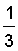+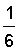= ?Solution: We need to make the denominators the same. We can find a common denominator by multiplying the denominators together: 3 x 6 = 18.  So instead of having 3 or 6 slices of pizza, we will make both of them have 18 slices. The pizzas now look like this: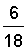+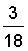=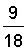In the problem above, we found a common denominator by multiplying the denominators of the original fractions. However, for most chefs, making 18 slices is too much work! Let's try using another method that involves less slices.

Method 2: We can rename these fractions using their least common denominator (LCD), which is the smallest number that is evenly divisible by all the denominators. It is the least common multiple of the denominators. Lets' find the LCD of one-third and one-sixth.List the multiplies of 3: 3, 6, 9, 12, 15, 18, 21,  ...List the multiplies of 6: 6, 12, 18, 24, 30,  ... List common multiples of 3 and 6: 6, 12, 18 List the least common multiple of 3 and 6: 6
 Solution: Now we can use 6 as our least common denominator.+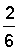=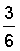As you can see, the least common denominator lets you add (or subtract) fractions using the least number of slices. It is not always practical to draw circles to solve these problems. So we need an arithmetic method. We will use equivalent fractions to help us, as shown in the examples below.

Example 1: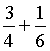Analysis:

The denominators are not the same. The least common denominator (LCD) of 4 and 6 is 12.

Solution: Make equivalent fractions with the new denominator: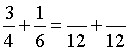and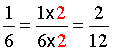In example 1, note that the numerator and the denominator of a fraction must be multiplied by the same nonzero whole number in order to have equivalent fractions. We could have used a common denominator, such as 24, to solve this problem. This is shown below.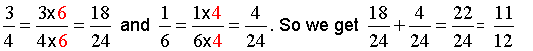As you can see, using a common denominator instead of the LCD can lead to unnecessary simplifying of the result (like having more slices of pizza). We have presented two methods for adding (and subtracting) fractions with unlike denominators:

1. Common denominators -- leads to having more slices of pizza.
2. Least common denominator (LCD) -- leads to having less slices of pizza.

You can use either method, whichever you prefer. However, for the remainder of this lesson, we will use the LCD method. Remember that the LCD is simply the least common multiple of the denominators. Let's look at some examples.

Example 2:Anaysis: The denominators are not the same. The LCD of 3 and 2 is 6.

Solution: Make equivalent fractionswith the new denominator: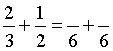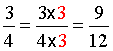and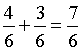Simply the result:In example 2, we had an improper fraction, so it was necessary to simplify the result. Let's look at some more examples.

Example 3: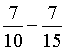Analysis: The denominators are not the same. The LCD of 10 and 15 is 30.

Solution: Make equivalent fractions with the new denominator: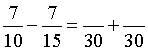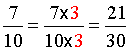and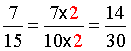Subtract the numerators: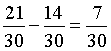Example 4:Analysis: The denominators are not the same. The LCD of 6, 8 and 16 is 48.

Solution: Make equivalent fractions with the new denominator: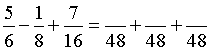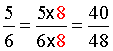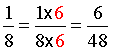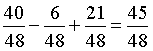Simply the result:The following procedure summarizes the steps we used in examples 1 through 4:

Procedure: To add or subtract fractions with unlike denominators:

1. Find the least common denominator.
2. Make equivalent fractions using the LCD.
3. Add or subtract the numerators.
4. Simplify the result if necessary.

For step 2, remember that the numerator and the denominator of a fraction must be multiplied by the same nonzero whole number in order to have equivalent fractions. Let's look at some word problems.Example 5: A member of the school track team ran two-thirds mile on Monday, and one-fifth mile on Tuesday. How many miles did he run altogether?

Analysis: This problem is asking us to add fractions with unlike denominators: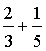Solution: The LCD of 3 and 5 is 15.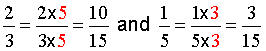Example 6: At a pie-eating contest, Spencer got through three-fourths of a pie before time was called; Carly finished just one-half of a pie. How much more pie did Spencer eat than Carly?

Analysis: This problem is asking us to subtract fractions with unlike denominators: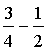Solution: The LCD of 4 and 2 is 4.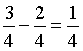Summary: In order to add or subtract fractions, they must have like denominators. Given two or more fractions with unlike denominators, the LCD is the least common multiple of the denominators.

To add or subtract fractions with unlike denominators

1. Find the least common denominator.
2. Make equivalent fractions using the LCD.
3. Add or subtract the numerators.
4. Simplify the result if necessary.

### Exercises

Directions: Add the fractions in each exercise below. Be sure to simplify your result, if necessary. Click once in an ANSWER BOX and type in your answer; then click ENTER. After you click ENTER, a message will appear in the RESULTS BOX to indicate whether your answer is correct or incorrect. To start over, click CLEAR.

Note: To write the fraction three-fourths, enter 3/4 into the form. To write the mixed number four and two-thirds, enter 4, a space, and then 2/3 into the form.

 1.ANSWER BOX:   RESULTS BOX:
 2.ANSWER BOX:   RESULTS BOX:
 3.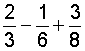ANSWER BOX:   RESULTS BOX:
 4. Maria's team practiced soccer for two-thirds of an hour on Friday, and for five-sixths of an hour on Saturday. How many hours of soccer did her team practice altogether? ANSWER BOX:   RESULTS BOX:
 5. Amy's history textbook weighs seven-eighths of a pound, and her algebra textbook weighs two-thirds of a pound. How much more does her history textbook weigh than her algebra textbook? ANSWER BOX:   RESULTS BOX:
 Lessons on Adding and Subtracting Fractions and Mixed Numbers 1. Adding Fractions with Like Denominators 2. Subtracting Fractions with Like Denominators 3. Adding and Subtracting Fractions with Unlike Denominators 4. Adding Mixed Numbers 5. Subtracting Mixed Numbers 6. Solving Word Problems 7. Practice Exercises 8. Challenge Exercises 9. Solutions